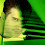## Friday, February 28, 2014

### Geometry Problem 988: Right Triangle, Cevians, Angles, 30 Degrees, Triple Angle

Level: Mathematics Education, High School, Honors Geometry, College.

Click the figure below to see the complete problem 9881.2.A slightly modified approach

Mark X on AD extended and Y on AC such that EA = EX = EY
Now E is the centre of circle AXY and so < XEY = 2 X < XAY = 60
Hence Tr. XEY is equilateral and EA = EX = EY = XY

Now < ADE = 3α – (60+ α) = 2α – 60 and so < XED = (2α – 60) – (α – 30) = α – 30 = < EAX
Therefore XE^2 = XD.XA = XY^2 and so < XYD = < XAY = 30

But < YCD = 90-α = < YXD hence CDXY is concyclic and so < DCX = 30 and hence XY = XC

It follows that Y is the centre of Tr. CEY and so x = < EXY = 60/2 = 30

Sumith Peiris
Moratuwa
Sri Lanka

3.Sumith, congratulations for your solution. Certainly difficult to find. Best Regads, Joaquim Maia, Brazil

1.Thank you Joaquim

Best Rgds

Sumith